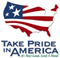# The National Flood-Frequency Program—Methods for Estimating Flood Magnitude and Frequency in Rural Areas in Arkansas

#### U.S. Geological Survey Fact Sheet 128-97 June, 1999

This report is available in pdf 714KB.

# Introduction

Estimates of the magnitude and frequency of flood-peak discharges and flood hydrographs are used for a variety of purposes, such as for the design of bridges, culverts, and flood-control structures; and for the management and regulation of flood plains. To provide simple methods of estimating flood-peak discharges, the U.S. Geological Survey (USGS) has developed and published equations for every State, the Commonwealth of Puerto Rico, and a number of metropolitan areas in the United States. In 1993, the USGS, in cooperation with the Federal Emergency Management Agency and the Federal Highway Administration, compiled all current USGS statewide and metropolitan area equations into a computer program, titled “The National Flood-Frequency (NFF) Program” (Jennings and others, 1994).

Since 1993, new or updated equations have been developed by the USGS for various areas of the Nation. These new equations have been incorporated into an updated version of the NFF Program.

Fact sheets that describe application of the updated NFF Program to various areas of the Nation are available. This fact sheet describes the application of the updated NFF Program to streams that drain rural areas in Arkansas.

## Overview

Arkansas is divided into four hydrologic regions (fig. 1) on the basis of drainage boundaries and physiography. Hodge and Tasker (1995) developed regression equations for estimating peak discharges (QT), in cubic meters per second, that have recurrence intervals (T) that range from 2 to 500 years for ungaged, unregulated, rural streams with drainage areas of less than 7,770 square kilometers.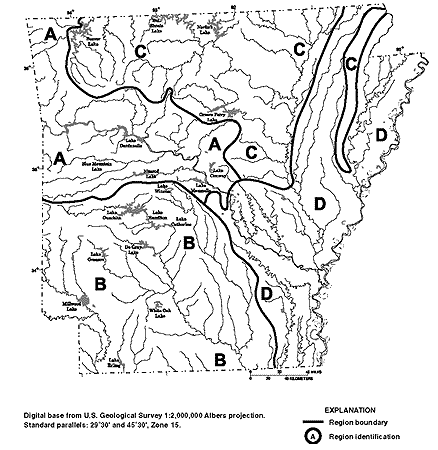Figure 1. Hydrologic regions for Arkansas

## Procedure

The updated equations presented in this report are in metric units; however, the NFF Program will accept input and report results in either the inch-pound or the metric system of units. The explanatory watershed variables used in the regression equations are as follow:

Drainage area (A), in square kilometers, is the total area that contributes runoff upstream of the stream site of interest.

Main channel slope (S), in meters per kilometer, is the difference in elevations at points 10 and 85 percent of the distance along the main channel (measured upstream from the location of the desired peak discharge to the drainage divide) divided by the distance between the points.

Mean basin elevation (E), in meters above sea level [National Geodetic Vertical Datum of 1929 (NGVD of 1929)], is the mean elevation of the drainage basin determined from topographic maps by the grid-sampling method. The grid-sampling method averages the point value of the variable in question at approximately 50 regularly spaced nodes across the drainage area.

Basin shape factor (SH), a dimensionless number, is the drainage area divided by the square of the main channel length.

The main channel length, in kilometers, is the distance between the gaged site and the basin divide (used in computation of the basin shape factor only).

The regression equations, the average standard errors of prediction, and the equivalent years of record are shown in table 1. The average standard errors of prediction are an average measure of the accuracy of the regression equations when estimating peak-discharge values for ungaged watersheds similar to those that were used to derive the regression equations. The equivalent years of record is the number of years of streamflow record needed to achieve the same accuracy as the regression equation. The ranges of the explanatory watershed variables over which the equations are applicable are shown in table 2. The standard error of prediction increases appreciably when any explanatory watershed variable is outside the quoted range of data used to develop the equations.

Regression equation Average standard error Equivalent years of record or prediction, in percent Table 1. Flood-peak discharge regression equations and associated statistics for streams that drain rural areas in Arkansas (modified from Hodge and Tasker, 1995) [QT, peak discharge for recurrence interval T, 2 to 500 years, in cubic meters per second; A, drainage area, in square kilometers; S, main channel slope, in meters per kilometer; E, mean basin elevation, in meters above sea level (NGVD of 1929); SH, basin shape factor] Q2 = 1.88A0.795S0.113 42 4 Q5 = 3.26A0.802S0.157 32 7 Q10 = 4.31A0.804S0.178 31 10 Q25 = 5.74A0.804S0.197 32 13 Q50 = 6.89A0.808S0.209 34 14 Q100 = 8.05A0.803S0.210 37 14 Q500 = 10.9A0.803S0.241 45 13 Q2 = 0.041A0.745E0.927SH0.342 42 4 Q5 = 0.113A0.735E0.875SH0.396 39 5 Q10 = 0.182A0.729E0.854SH0.422 38 7 Q25 = 0.288A0.723E0.839SH0.446 38 9 Q50 = 0.377A0.718E0.832SH0.460 39 11 Q100 = 0.471A0.715E0.827SH0.472 40 12 Q500 = 0.714A0.708E0.823SH0.494 43 14 Q2 = 4.18A0.669 42 3 Q5 = 7.23A0.676 38 5 Q10 = 9.44A0.680 37 6 Q25 = 12.3A0.684 36 9 Q50 = 14.6A0.687 35 11 Q100 = 16.7A0.690 35 12 Q500 = 22.1A0.694 37 15 Q2 = 2.56A0.667S0.211SH0.208 33 3 Q5 = 4.16A0.688S0.289SH0.252 31 4 Q10 = 5.25A0.699S0.334SH0.270 33 4 Q25 = 6.67A0.710S0.385SH0.286 37 4 Q50 = 7.72A0.717S0.418SH0.295 41 4 Q100 = 8.76A0.723S0.448SH0.302 45 4 Q500 = 11.2A0.735S0.510SH0.316 56 4

Hydrologic study region Drainage area, in square kilometers Main channel slope, in meters per kilometer Mean basin elevation, in meters above sea level1 Basin shape factor Table 2. Range of explanatory variables for which regression equations are applicable [--, not applicable] Region A 0.23-1,980 0.56-70.3 -- -- Region B 0.13–6,890 -- 43.3–381 0.058–0.642 Region C 0.21–5,280 -- -- -- Region D 0.52–4,530 0.086–7.73 -- 0.015–0.533

The regression equations were developed by using peak-discharge data for streamflow-gaging stations with at least 10 years of record collected at 189 locations in Arkansas and 15 locations in Missouri, Oklahoma, and Louisiana.

## Improving Estimates with Gaged Data

The U.S. Water Resources Council (1981, appendix 8) described, and Hodge and Tasker (1995) applied, weighting techniques to improve estimates of peak discharge at gaged locations. The improved estimates are obtained by combining the estimates derived from analysis of gage records with estimates derived by other means, including regression equations. The weights of the two independent estimates are based on the length of the gage record (in years) and the equivalent years of record of the applicable regression equation. The weighted estimate of peak discharge is computed as: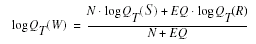where

 QT(W) is the weighted estimate for recurrence interval T at the gaged site, QT(S) is the estimate of QT derived from analysis of the gage records, QT(R) is the estimate of QT derived from application of the regression equation, N is the number of years of gage record, and EQ is the equivalent years of record (table 1).

The accuracy of the weighted discharge estimate, in equivalent years of record, is equal to N + EQ. The NFF Program contains algorithms for the weighting computations, which differ slightly from the equation described by Hodge and Tasker (1995).

Ungaged Sites Near Gaged Sites on the Same Stream
Hodge and Tasker (1995) showed how the weighted estimate for peak discharge at a gaged site can be used to improve estimates of peak discharge for an ungaged site on the same stream that has a drainage that is between 50 and 150 percent of the drainage area of the gaged site. The regression estimate for the ungaged site is multiplied by an adjustment factor, which is computed as: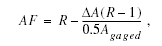where

 AF is the adjustment factor,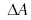is the difference in drainage area (Agaged -Aungaged), and R is the number of years of gage record, and EQ is the ratio of the weighted peak-discharge estimate at the gaged site to the regression estimate at the ungaged site, QT(W)/QT(R).

The equations are used without adjustment where the drainage area of the ungaged site is not within 0.5 to 1.5 times the drainage area of the gaged site.

## Sites in Transition Zones

When the drainage area of the site of interest is in more than one region, a weighted estimate of the peak discharge should be computed. The equations for the appropriate regions should be applied independently using basinwide estimates of the required explanatory variables as if the entire basin was in each region. The weighted estimate is then computed by multiplying each regional estimate against the fraction of the drainage area in that region and summing the products. The NFF Program provides an algorithm for this computation.

Estimates of peak discharge (QT) derived from gaged records and the lengths of the gaged records (N) are summarized by Hodge and Tasker (1995). They also presented annual peak-flow data, and explanatory watershed variables for the 204 streamflow-gaging stations used to develop the regression equations. Hodge and Tasker (1995) did not include data from large main stem stations on the Red, the Arkansas, the White, the Black, the St. Francis, the Mississippi, and the Ouachita Rivers in the regression analysis because of their unique, large river floodflow characteristics.

## Region of Influences Technique

Hodge and Tasker (1995) included an additional regression analysis that is based on the region of influence regression technique. The region of influence regression technique generates an independent regression equation for a site by analyzing data from a set of sites with similar explanatory watershed characteristics. The sites included in the set are not necessarily in the same hydrologic region as the site in question. On the basis of a root-mean-square error comparison, Hodge and Tasker (1995) concluded that the independent regression equations based on the region of influence regression technique outperforms the regional regression equations in regions A, B, and C; the regional regression equations are more accurate in region D. Hodge and Tasker (1995) stated, “The region of influence method is still being improved and is to be considered only as a second alternative to the regional regression equations.”

## References

Hodge, S.A., and Tasker, G.D., 1995, Magnitude and frequency of floods in Arkansas: U.S. Geological Survey Water-Resources Investigations Report 95-4224, 52 p.

Jennings, M.E., Thomas, W.O., Jr., and Riggs, H.C., comps., 1994, Nationwide summary of U.S. Geological Survey regional regression equations for estimating magnitude and frequency of floods for ungaged sites, 1993: U.S. Geological Survey Water-Resources Investigations Report
94–4002, 196 p.

U.S. Water Resources Council, 1981, Guidelines for determining flood flow frequency: U.S. Water Resources Council Bulletin 17B, 28p. 14 appendixes.

U.S. Geological Survey
Office of Surface Water
415 National Center
Reston, Virginia 20192
(703) 648-5301

USGS hydrologic analysis software is available for electronic retrieval through the World Wide Web (WWW) at: http://water.usgs.gov/software/ and through anonymous File Transfer Protocol (FTP) from water.usgs.gov (directory: /pub/software). The WWW page and FTP directory from which the National Flood-Frequency software and user documentation can be retrieved are http://water.usgs.gov/software/nff.html and /pub/software/surface_water/nff, respectively.

Additional earth science information is available from the USGS through the WWW at http://www.usgs.gov/ anonymous or by calling 1-888-ASK-USGS.

 AccessibilityFOIAPrivacyPolicies and Notices U.S. Department of the Interior, U.S. Geological Survey Persistent URL: Page Contact Information: Contact USGS Last modified: Tuesday, November 29 2016, 05:53:52 PM